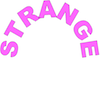#### You may also like### Gaxinta

A number N is divisible by 10, 90, 98 and 882 but it is NOT divisible by 50 or 270 or 686 or 1764. It is also known that N is a factor of 9261000. What is N?### Thirty Six Exactly

The number 12 = 2^2 × 3 has 6 factors. What is the smallest natural number with exactly 36 factors?### Strange Numbers

All strange numbers are prime. Every one digit prime number is strange and a number of two or more digits is strange if and only if so are the two numbers obtained from it by omitting either its first or its last digit. Find all strange numbers.

# Stars

##### Age 11 to 14Challenge Level

Stars printable sheet
There are printable circles of dots on the NRICH Printable Resources page, under Circle templates > Without central point

Draw some stars using the interactivity below.
Choose how many points you would like around your circle
Now choose a starting point and drag to another point. Watch as the pattern continues to travel around the circle...

When a circle has 8 dots you can move around the circle in steps of length $1$, $2$, $3$, $4$, $5$, $6$ or $7$.

If you move around the circle in steps of $2$, you miss some points

If you move around the circle in steps of $3$, you visit all the points.

How else can you visit all the points?

When a circle has $9$ dots there are $6$ different step sizes where you visit every point.

Which step sizes allow you to do this?

Now consider $10$ points. Can you find the $4$ different step sizes in which we can visit every point?

Explore what happens with different numbers of points and different step sizes.

How can you work out what step sizes will visit all the points for any given number of points?

Now consider $5$ points. You can visit all the points irrespective of the step size. Which other numbers have this property?

Can you find a relationship between the number of dots on the circle and the number of steps that will ensure that all points are hit?

If you would rather work on paper, go to the Printable Resources page to open PDF files of the circles (Circle templates > Without central point)

Each PDF file contains 12 identical circles with a specific number of dots. You can select any number of dots from 3 to 24.